Monomials, Binomials, Trinomials

Chapter 10 Class 7 Algebraic Expressions
Concept wise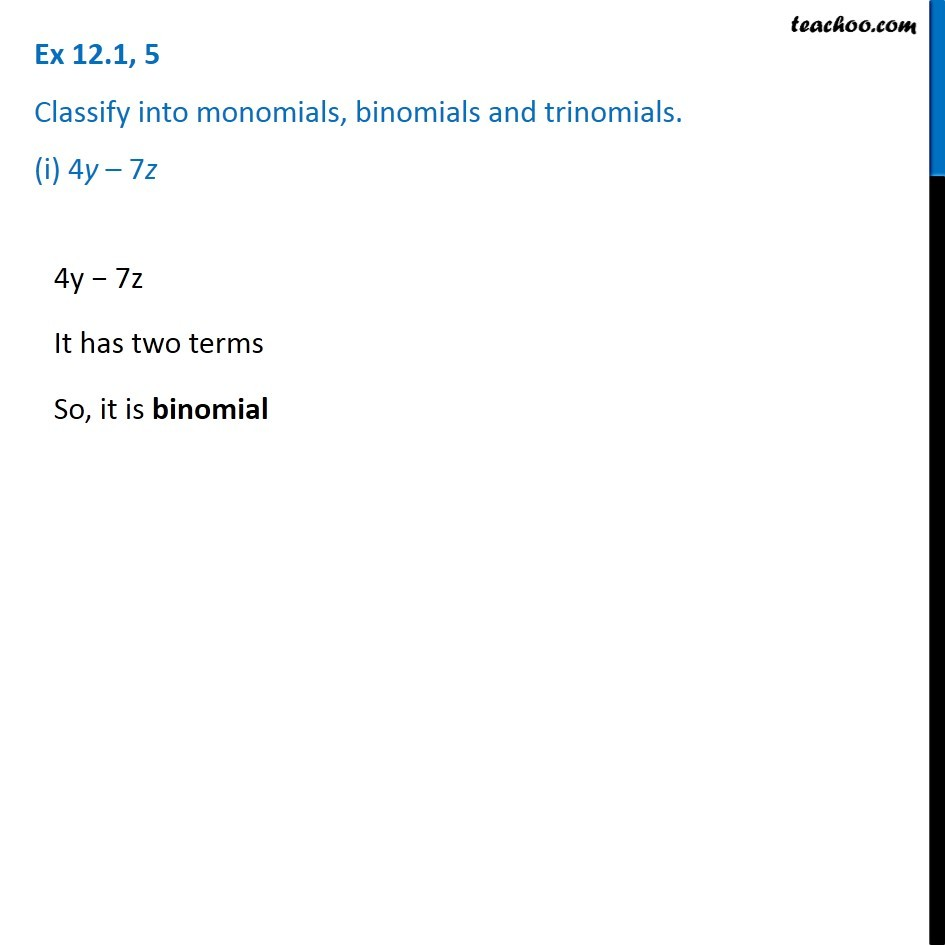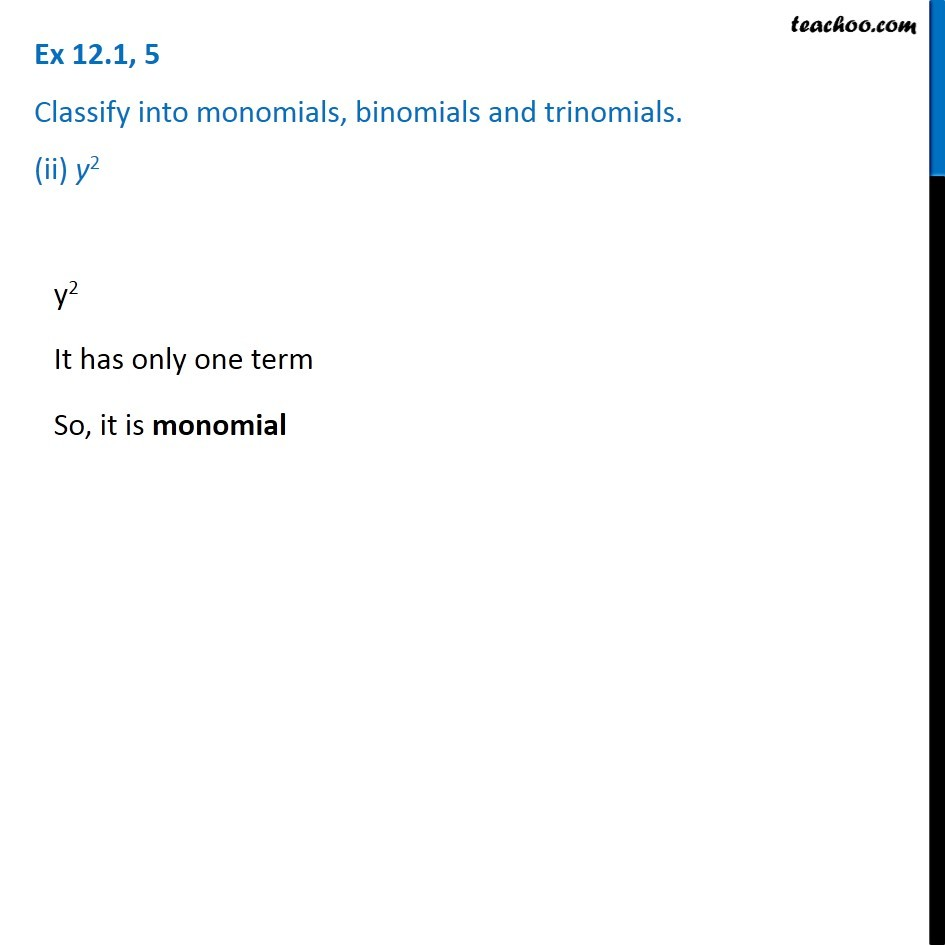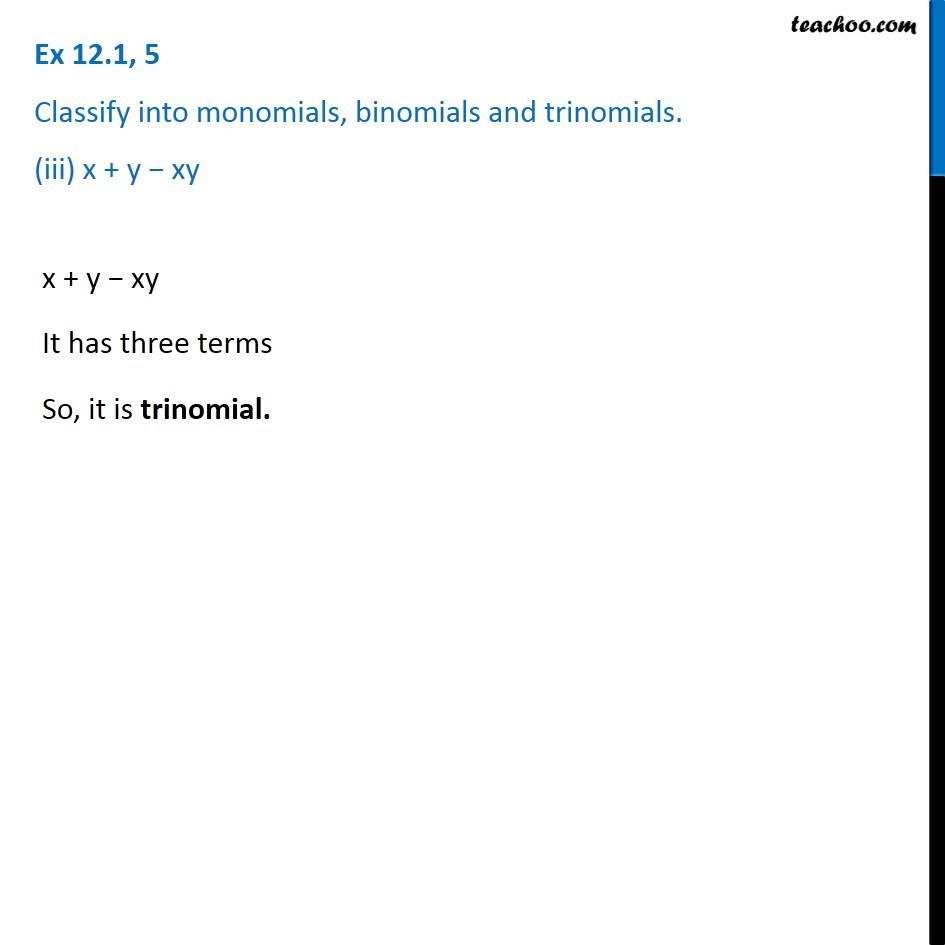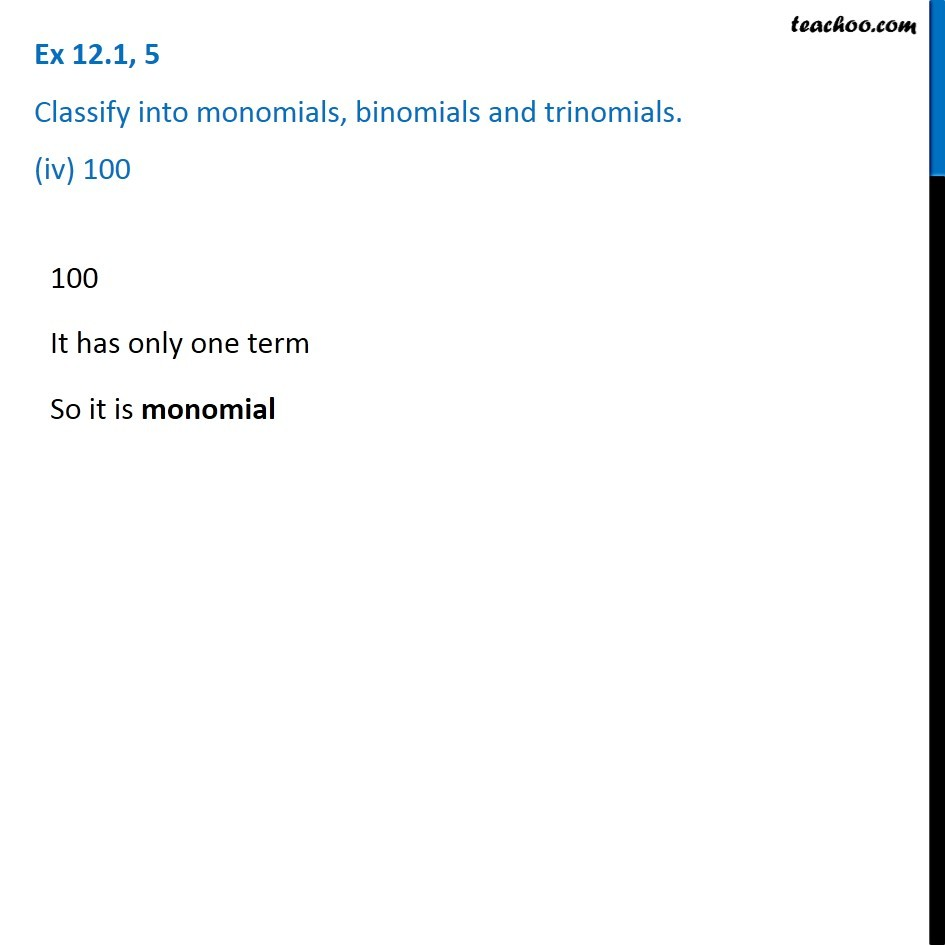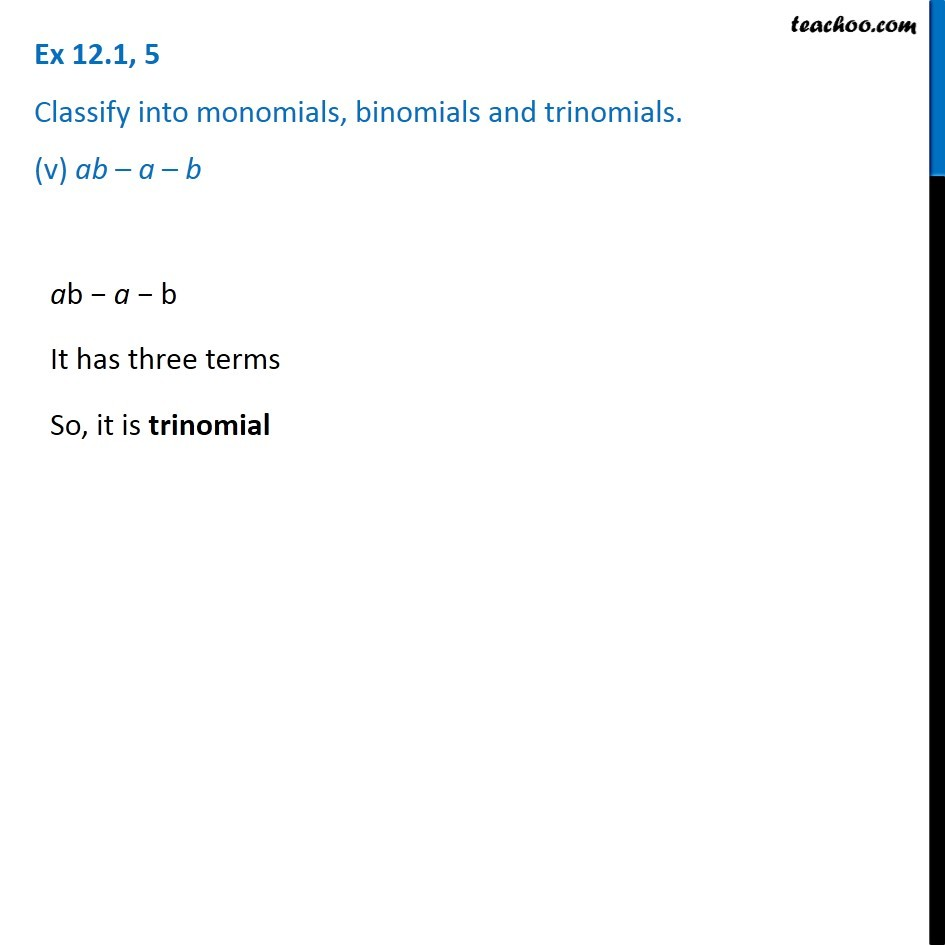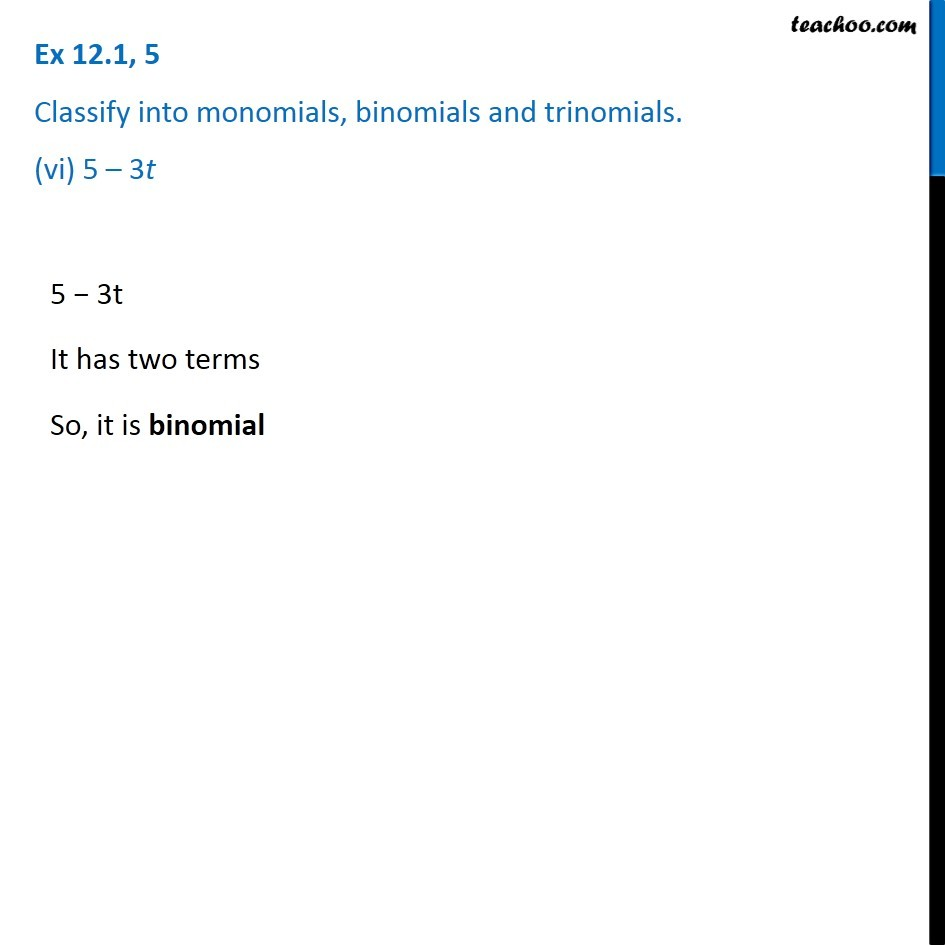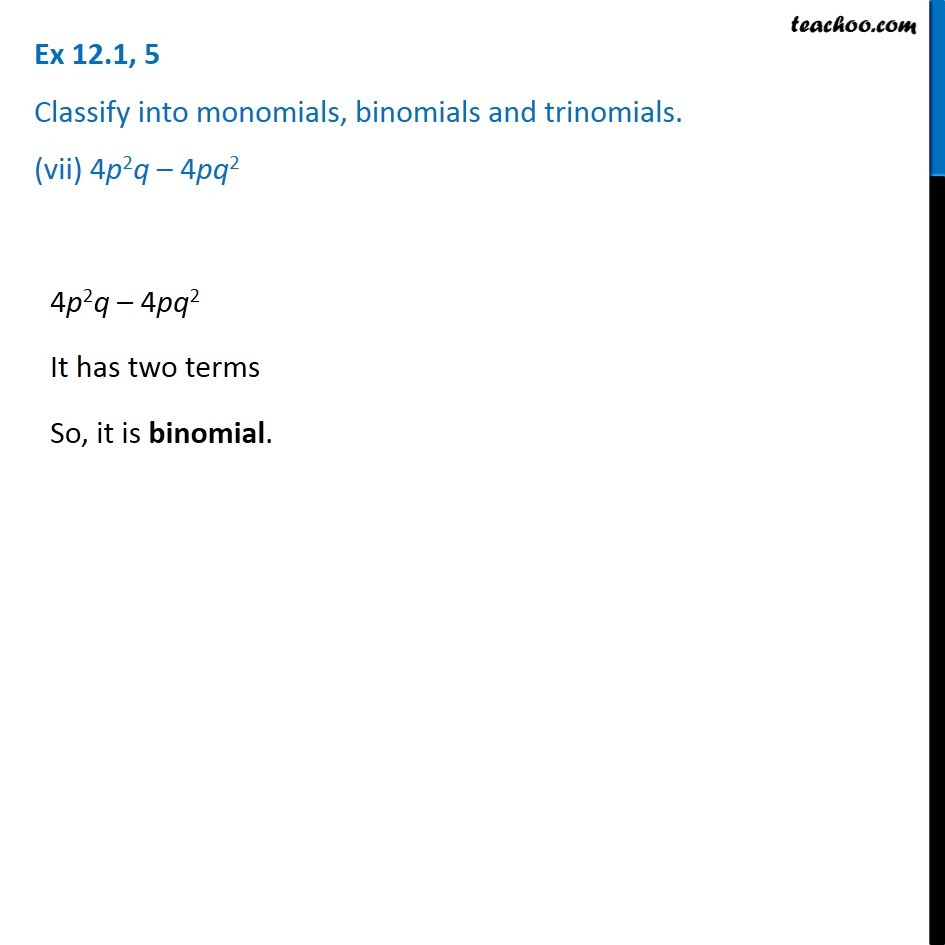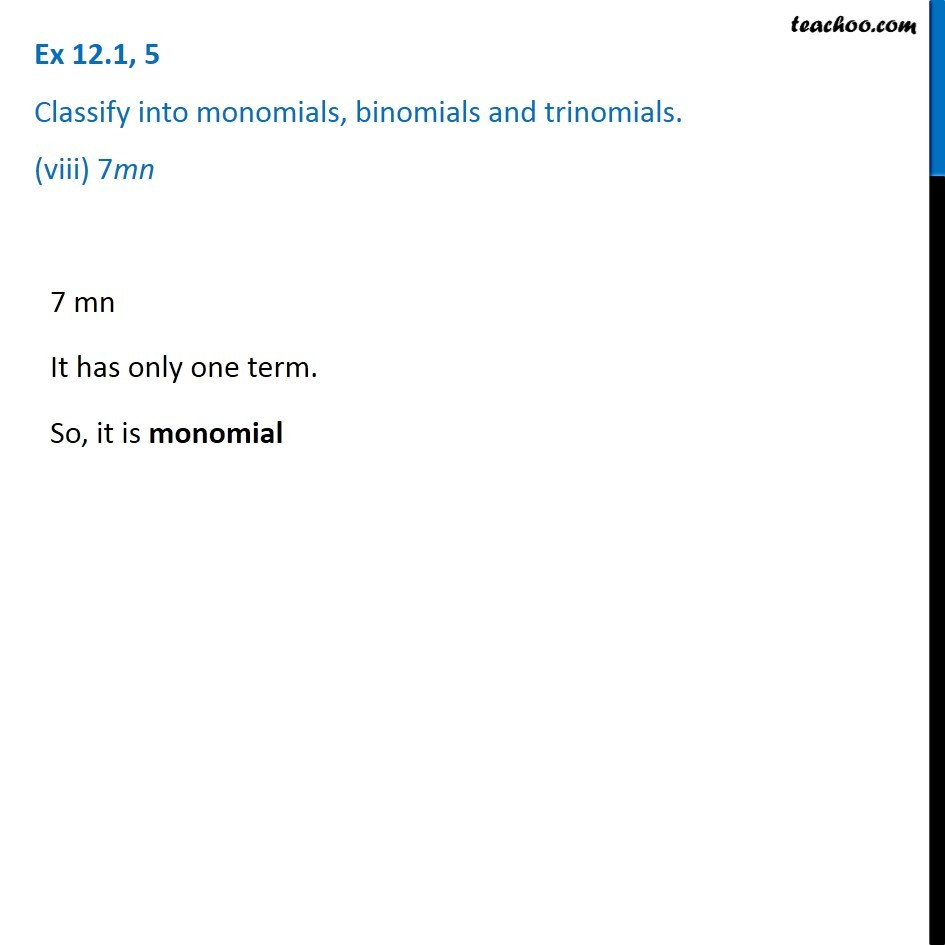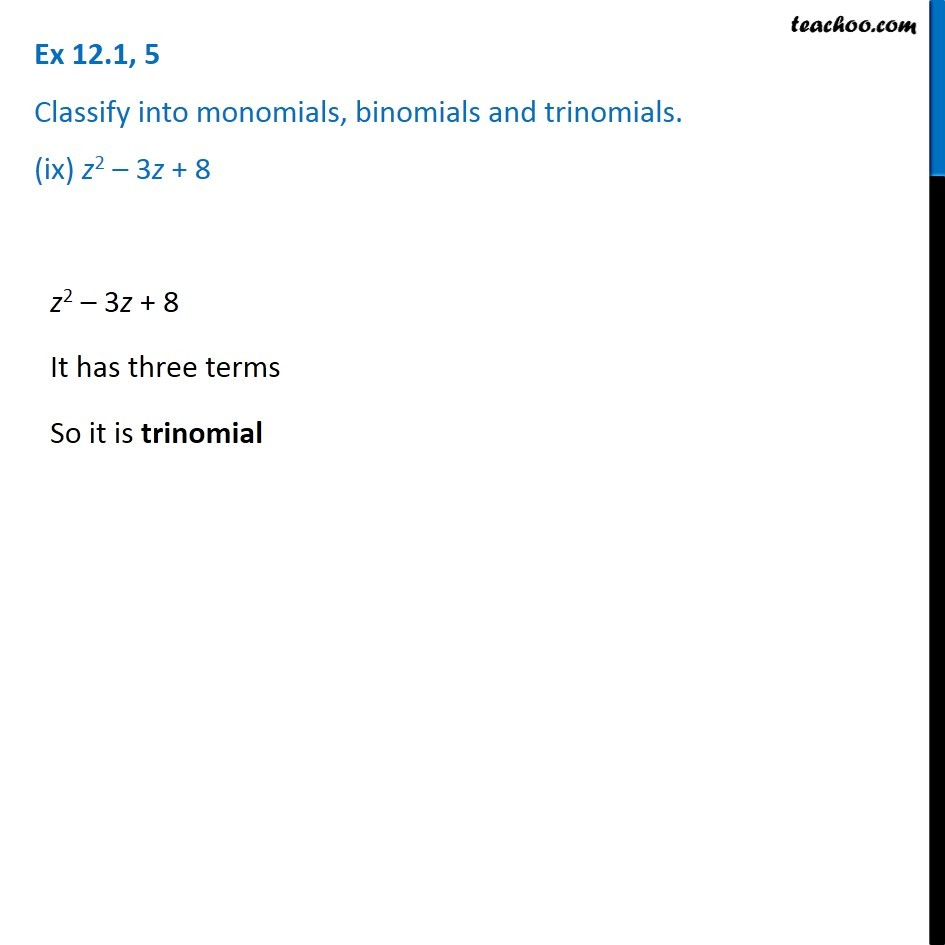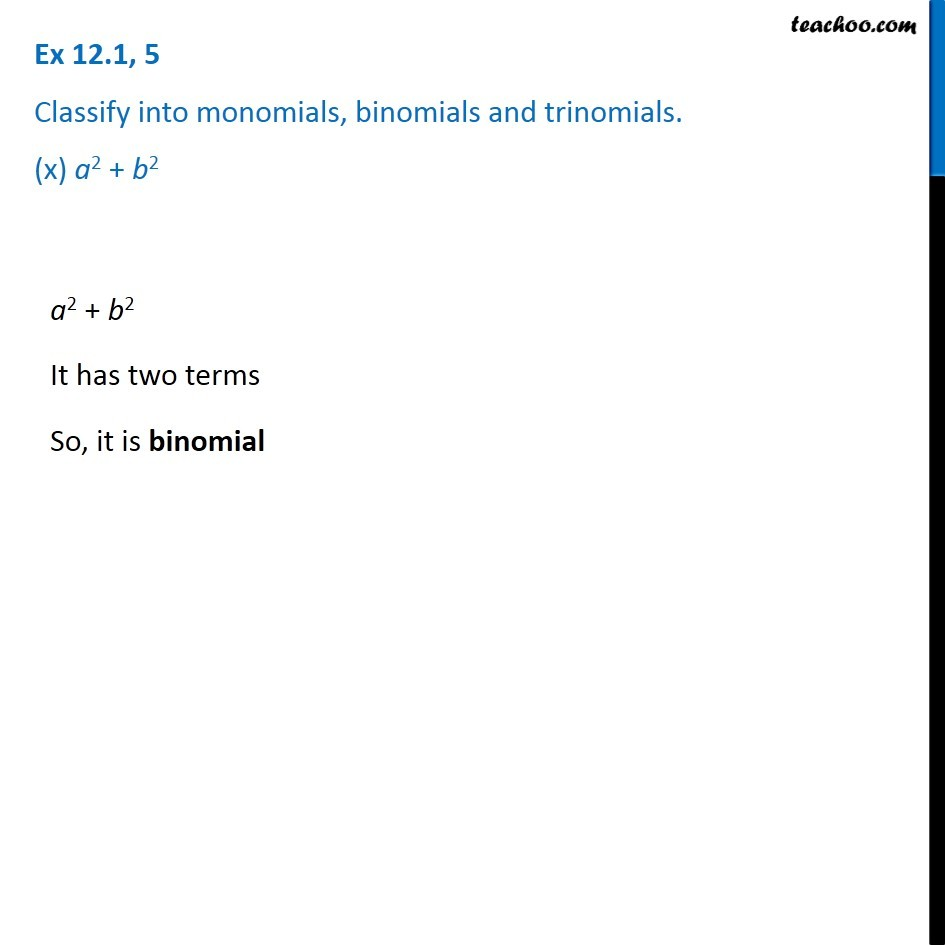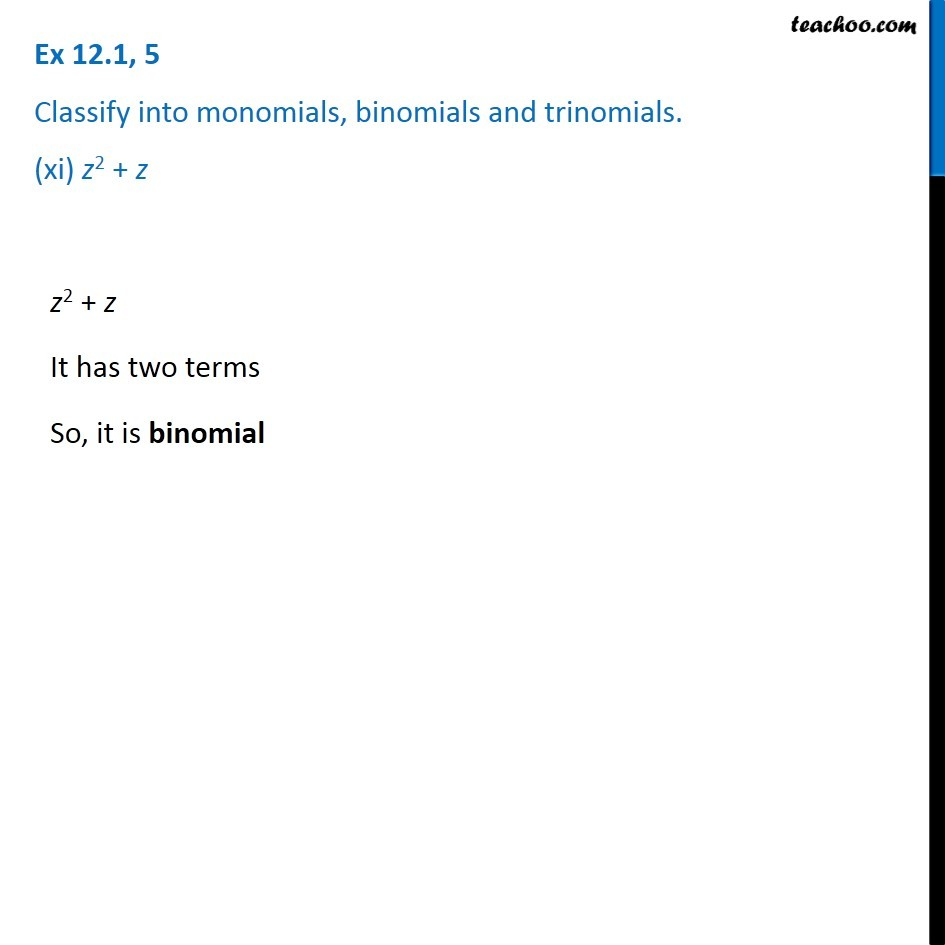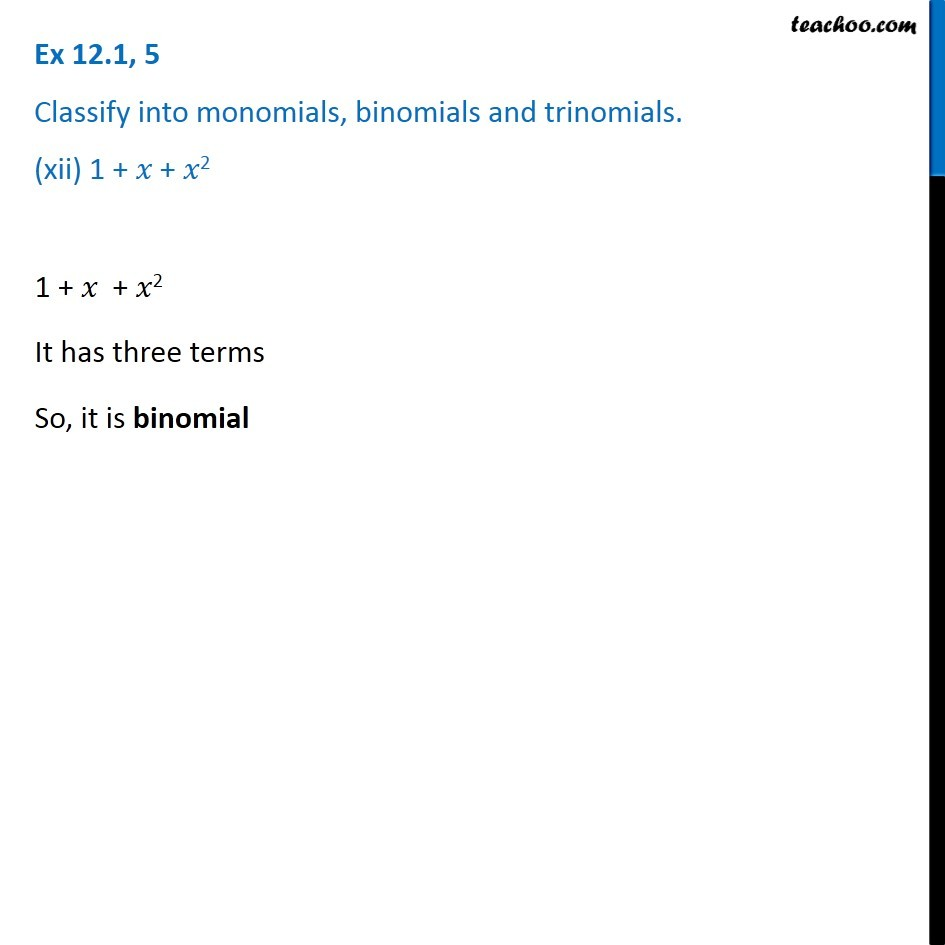Learn in your speed, with individual attention - Teachoo Maths 1-on-1 Class

### Transcript

Ex 10.1, 5 Classify into monomials, binomials and trinomials. (i) 4y – 7z 4y − 7z It has two terms So, it is binomial y2 It has only one term So, it is monomial Ex 10.1, 5 Classify into monomials, binomials and trinomials. (iii) x + y − xy x + y − xy It has three terms So, it is trinomial. Ex 10.1, 5 Classify into monomials, binomials and trinomials. (iv) 100 100 It has only one term So it is monomial ab − a − b It has three terms So, it is trinomial Ex 10.1, 5 Classify into monomials, binomials and trinomials. (vi) 5 – 3t 5 − 3t It has two terms So, it is binomial Ex 10.1, 5 Classify into monomials, binomials and trinomials. (vii) 4p2q – 4pq2 4p2q – 4pq2 It has two terms So, it is binomial. Ex 10.1, 5 Classify into monomials, binomials and trinomials. (viii) 7mn 7 mn It has only one term. So, it is monomial Ex 10.1, 5 Classify into monomials, binomials and trinomials. (ix) z2 – 3z + 8 z2 – 3z + 8 It has three terms So it is trinomial Ex 10.1, 5 Classify into monomials, binomials and trinomials. (x) a2 + b2 a2 + b2 It has two terms So, it is binomial Ex 10.1, 5 Classify into monomials, binomials and trinomials. (xi) z2 + z z2 + z It has two terms So, it is binomial